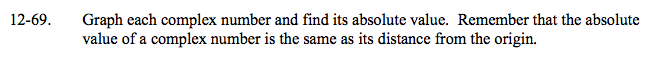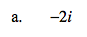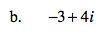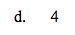### Home > A2C > Chapter 12 > Lesson 12.1.4 > Problem12-69

12-69.
1. Graph each complex number and find its absolute value. Remember that the absolute value of a complex number is the same as its distance from the origin. Homework Help ✎

1. −2i

2. −3 + 4i

3. −2 − 2i

4. 4How far is this point away from 0 on your graph?

The absolute value is 2.How far is this point away from 0 on your graph? The Pythagorean Theorem will be helpful here.

The absolute value is 5.Look at parts (a) and (b).Looks at parts (a) and (b).